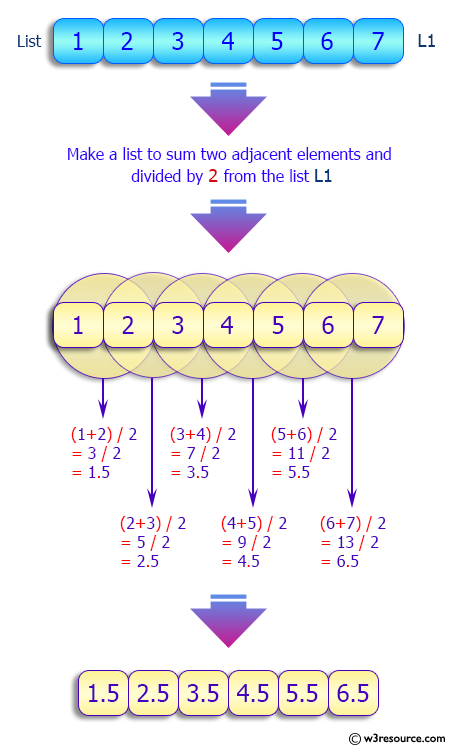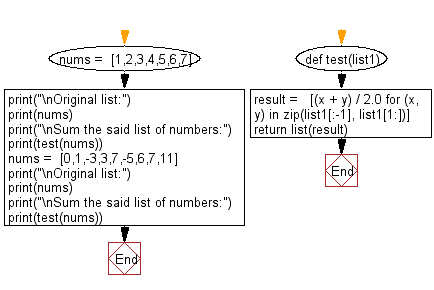﻿ Python: Sum a list of numbers - w3resource# Python: Sum a list of numbers

## Python List: Exercise - 208 with Solution

Sum a list of numbers. Write a Python program to sum the first number with the second and divide it by 2, then sum the second with the third and divide by 2, and so on.
Equation: (element 0 + element 1) / 2, (element 1 + element 2) / 2, ... etc.

Sample Solution:

Python Code:

``````#Source: shorturl.at/csxLM
def test(list1):
result =   [(x + y) / 2.0 for (x, y) in zip(list1[:-1], list1[1:])]
return list(result)

nums =  [1,2,3,4,5,6,7]
print("\nOriginal list:")
print(nums)
print("\nSum the said list of numbers:")
print(test(nums))

nums =  [0,1,-3,3,7,-5,6,7,11]
print("\nOriginal list:")
print(nums)
print("\nSum the said list of numbers:")
print(test(nums))
```
```

Sample Output:

```Original list:
[1, 2, 3, 4, 5, 6, 7]

Sum the said list of numbers:
[1.5, 2.5, 3.5, 4.5, 5.5, 6.5]

Original list:
[0, 1, -3, 3, 7, -5, 6, 7, 11]

Sum the said list of numbers:
[0.5, -1.0, 0.0, 5.0, 1.0, 0.5, 6.5, 9.0]
```

Pictorial Presentation:Flowchart:## Visualize Python code execution:

The following tool visualize what the computer is doing step-by-step as it executes the said program:

Python Code Editor:

Have another way to solve this solution? Contribute your code (and comments) through Disqus.

What is the difficulty level of this exercise?

Test your Python skills with w3resource's quiz

﻿

## Python: Tips of the Day

Floor Division:

When we speak of division we normally mean (/) float division operator, this will give a precise result in float format with decimals.

For a rounded integer result there is (//) floor division operator in Python. Floor division will only give integer results that are round numbers.

```print(1000 // 300)
print(1000 / 300)```

Output:

```3
3.3333333333333335```